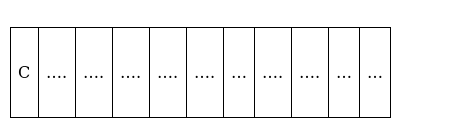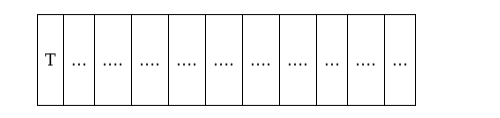# How many arrangements can be made by using all the letters of the word

Question:

(i)How many arrangements can be made by using all the letters of the word ‘MATHEMATICS’?

(ii) How many of them begin with C?

(iii) How many of them begin with T?

Solution:

(i) There are 11 letters of which 2 are of 1 kind, 2 are of another kind, 2 are of the 3rd kind

Total number of arrangements $=\frac{11 !}{2 ! 2 ! 2 !}=4989600$

(ii)There are 10 spaces to be filled by 10 letters of which 2 are of 3 different kinds

Number of arrangements $=\frac{10 !}{2 ! 2 ! 2 !}=453600$

(iii)There are 10 spaces to be filled by 10 letters of which 2 are of 2 different kinds

Number of arrangements $=\frac{10 !}{2 ! 2 !}=907200$